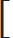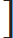# Number System : Arithmetic Progression and Geometric Progression

Hello Friends!

Under this post " Number System: Arithmetic Progression and Geometric Progression " we will see arithmetic progression and geometric progression, formula used for AP and GP and types of questions asked in competitive exams.

1) Geometric Progression / Geometric Series / Geometric Sequence : x, xr, xr2xr3, xr4, --- are said to be in geometric progression. Here, a is first term and r is common ratio.

a) nth term = xr (n -1)

 b) Sum of n terms = x (1 – rn) , here r < 1 (1 – r)
 c) Sum of n terms = x (rn – 1) , here r > 1 (r – 1)

2) Arithmetic Progression / Arithmetic Series / Arithmetic Sequence : x, x + y, x + 2y, x + 3y are said to be in A.P. Here x is first term and common difference is y.

a) nth term = x + (n – 1) y
 b) Sum of n terms = n [2x + (n – 1)y] 2

 3) 1 + 2 + 3 +- - - - + n = n(n + 1) 2

 4) (12 + 22 + 32 - - - - + n2) = n(n + 1) (2n + 1) 6

 5) (13 + 23 + 33 - - - - + n3) =n(n + 1)2 2
At last, A special thanks to choose LOUD STUDY.

If you have any doubts or confusion, please comment in the comment box below, we will try to reply you as soon as possible.